This week I split my time between wrapping up a book chapter on abstractive summarisation, trying to get UDA to work, and getting my hands dirty with movement pruning.

## A first look at movement pruning

This week I focused on a compression technique for Transformers called pruning, whose goal is to selectively delete the weights of a model according to some importance criterion. In particular I wanted to understand how movement pruning1 worked and how I could adapt Victor Sanh's implementation to run in Jupyter notebooks with the Trainer API from transformers.

The basic idea behind movement pruning is to gradually remove weights during fine-tuning such that the model becomes progressively sparser. As the authors observe, this "fine-pruning" approach addresses one of the main problems with other approaches like magnitude pruning that are designed for pure supervised learning tasks:

While magnitude pruning is highly effective for standard supervised learning, it is inherently less useful in the transfer learning regime. In supervised learning, weight values are primarily determined by the end-task training data. In transfer learning, weight values are mostly predetermined by the original model and are only fine-tuned on the end task. This prevents these methods from learning to prune based on the fine-tuning step, or “fine-pruning.”

Mathematically, the way most pruning methods work is to calculate a matrix ${\bf S}$ of importance scores and then select the top-$v$ percent of weights by importance:\mathrm{Top}_v({\bf S})_{ij} = \left\{ \begin{aligned} 1 && \mathrm{if} \, S_{ij} \mathrm{ \,in\, top\, } v\% \\ 0 && \mathrm{otherwise}\end{aligned} \right. From these scores we can then define a mask ${\bf M} \in \{0,1\}^{n\times n}$ that masks the weights during the forward pass with some input $x_i$ and effectively creates a sparse network:

$$a_i = W_{ik}M_{ik}x_k \,.$$

For example, magnitude pruning calculates the scores according to the magnitude of the weights ${\bf S} = \left(\mid W_{ij} \mid\right)_{1\leq j, j\leq n}$ and then the masks are derived from ${\bf M} = \mathrm{Top}_v({\bf S})$.

The key novelty with movement pruning is that both the weights and the scores are learned during fine-tuning. This implies that in the backward pass, we also track the gradient of the loss ${\cal L}$ with respect to $S_{ij}$:2

$$\frac{\partial{\cal L}}{\partial S_{ij}} = \frac{\partial {\cal L}}{\partial a_i}\frac{\partial a_i}{\partial S_{ij}} = \frac{\partial {\cal L}}{\partial a_i}W_{ij}x_j$$

Once the scores are learned, it is then straightforward to generate the mask using ${\bf M} = \mathrm{Top}_v({\bf S})$. The authors also propose a "soft" version of movement pruning where instead of picking the top-$v$% of weights, one uses a global threshold $\tau$ to define the binary mask: ${\bf M} = ({\bf S} > \tau)$.

The paper has a nice visualisation of how the pretrained weights of BERT are pruned during fine-tuning and shows how magnitude pruning tends to make the pruning decision mostly on the basis of the pretrained weights (i.e. weights that have small absolute value during pre-training get pruned).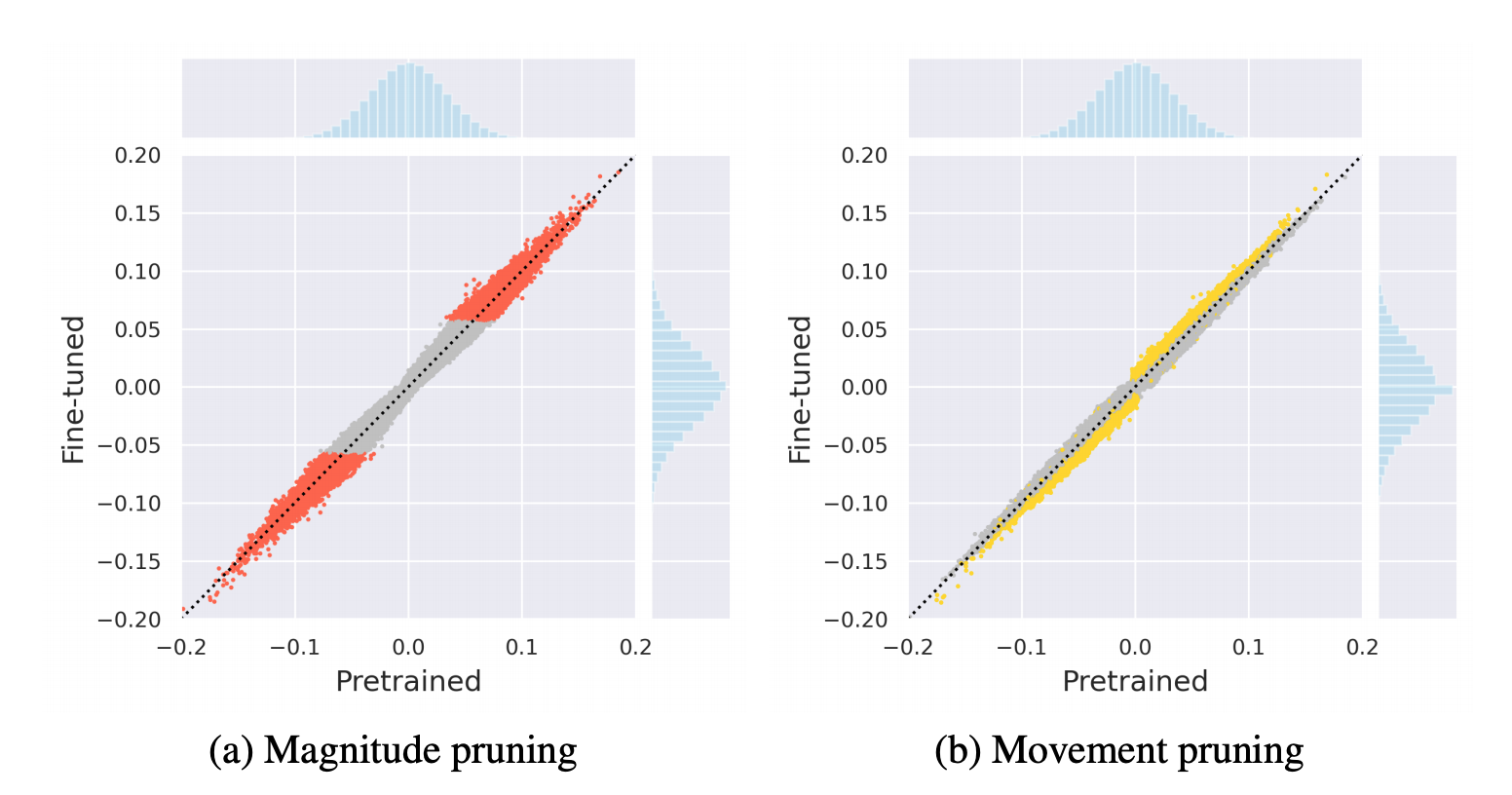In their experiments, the authors use a cubic sparsity scheduler to increase the amount of sparsity after some $t_i$ steps of warmp-up:

$$v^{(t)} = v_f + (v_i-v_f)\left(1 - \frac{t-t_i}{N\Delta t}\right)^3 \,.$$

The results for both hard and soft movement pruning on SQuAD and other benchmarks are quite impressive, especially in the high-sparsity regimes where less than 5% of the weights are retained!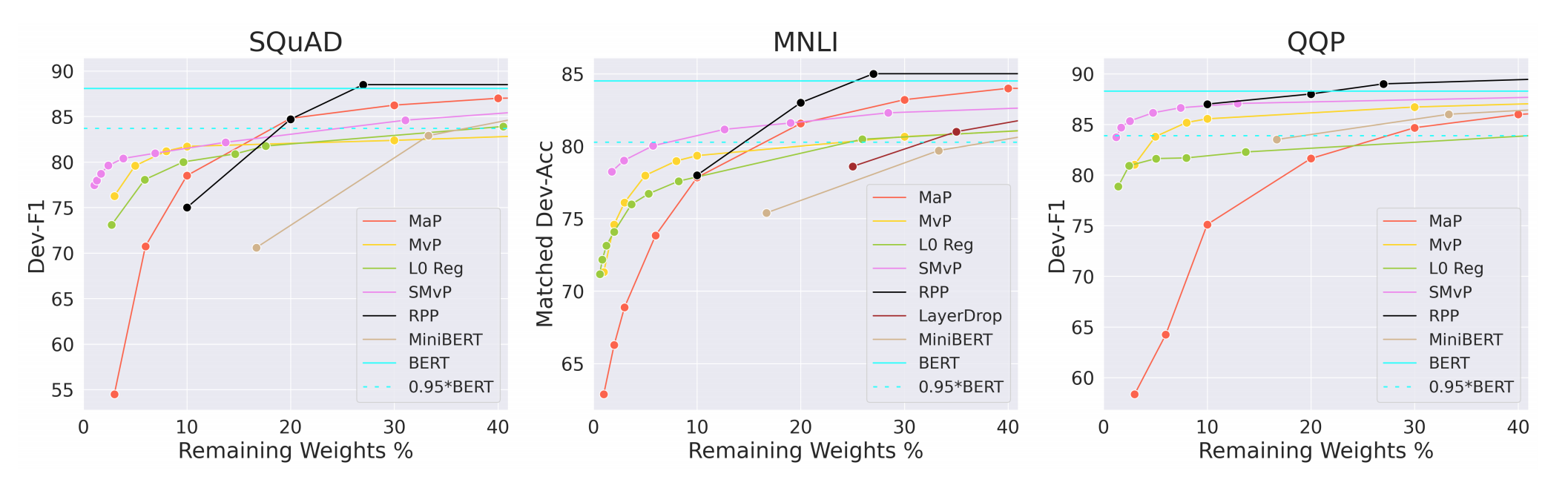## Implementing movement pruning

As noted above, I wanted to adapt Victor Sanh's implementation to work with the Trainer API from transformers so that I can run it in a Jupyter notebook. Implementing the Trainer itself was pretty straightforward and I was able to reuse a lot of Victor's code with minor adjustments. The first thing to do was override the compute_loss function as follows:

def compute_loss(self, model, inputs):
threshold, _ = self._schedule_threshold(...)
inputs["threshold"] = threshold
outputs = model(**inputs)
loss, _, _ = outputs
return loss


Here we use the sparsity scheduler to get the threshold value $\tau$ needed for soft movement pruning, add it to the inputs and then extract the loss from the forward pass. The next step was to override the create_optimizer_and_scheduler function to account for the fact that there is a learning rate $\alpha_S$ associated with calculating the scores matrix:

$$S_{ij}^{(T)} = -\alpha_S \sum_{t<T} \left( \frac{\partial {\cal L}}{\partial W_{ij}}\right)^{(t)} W_{ij}^{(t)}$$

In practice, this amounts to adding a term to the parameters we wish to optimize over

optimizer_grouped_parameters = [
{
"params": [p for n, p in self.model.named_parameters()
}, ...
]


so that the final function takes the form:

def create_optimizer_and_scheduler(self, num_training_steps):
no_decay = ["bias", "LayerNorm.weight"]
optimizer_grouped_parameters = [
{
"params": [p for n, p in self.model.named_parameters()
},
{
"params": [
p
for n, p in self.model.named_parameters()
and not any(nd in n for nd in no_decay)
],
"lr": self.args.learning_rate,
"weight_decay": self.args.weight_decay,
},
{
"params": [
p
for n, p in self.model.named_parameters()
and any(nd in n for nd in no_decay)
],
"lr": self.args.learning_rate,
"weight_decay": 0.0,
},
]

self.lr_scheduler = get_linear_schedule_with_warmup(
self.optimizer, num_warmup_steps=self.args.warmup_steps,
num_training_steps=self.t_total)


So far, so good ... but what I had not appreciated is that one needs special model classes to deal with sparse matrices! In Victor's implementation, this requires a wholescale rewrite of the BERT classes to replace all the torch.nn.Linear layers with a custom MaskedLinear layer and additional parameters to calculate the adaptive mask in the forward pass.

Although there is no plan to include these masked versions of BERT into the main transformers library, François Lagunas at HuggingFace pointed me to work he's done on making sparse matrices efficient in PyTorch.

In any case, I went ahead with Victor's masked models and ran a first set of experiments using 10% of the SQuAD data. To warmup, I used Victor's scripts as a benchmark and observed some peculiar features of fine-pruning: the metrics are flat for half the training before suddenly shooting up! Similarly, the loss gets worse before getting better. This is somewhat surprising, since fine-tuning usually gets most of the performance in the first 1-2 epochs of training before plateauing.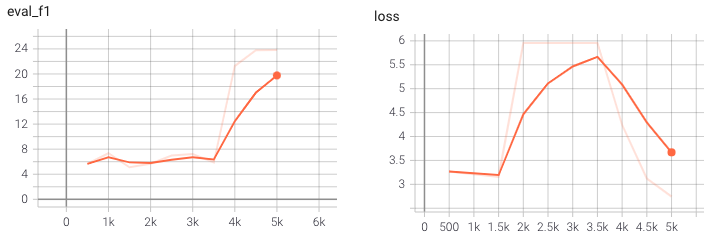So far I have not been able to reproduce these results in my implementation, with my model failing to recover from the charactersitic dip in performance during training: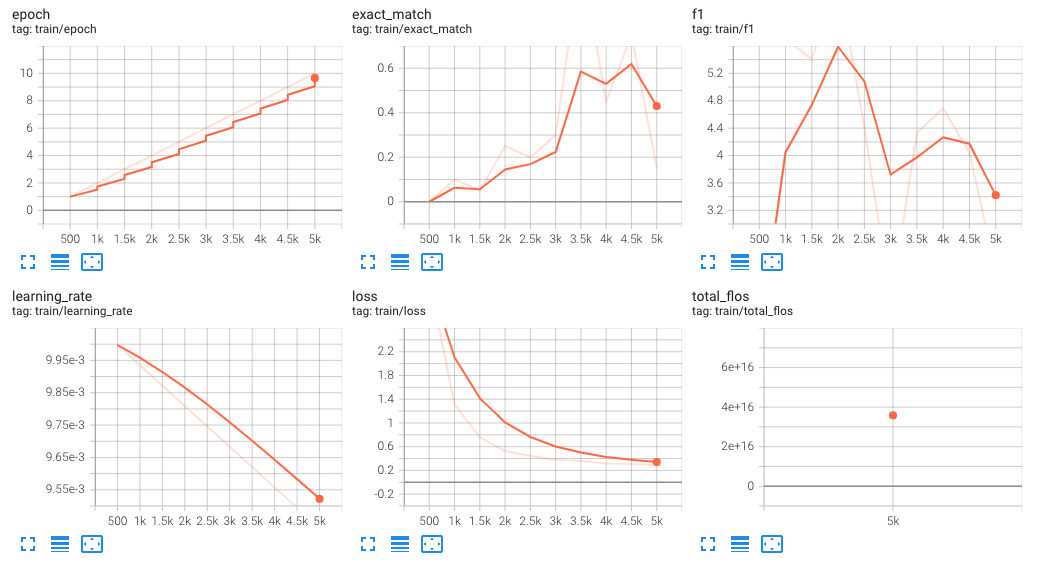So my focus for next week is to figure out what's going wrong and gradually scale-out to fine-pruning on the full SQuAD dataset!

## Universal data augmentation

For the last two weeks, Leandro von Werra and I have been dabbling in few-shot learning with Google's Unsupervised Data Augmentation for Consistency Training or UDA for short. For the book we're working on, one idea is to provide readers with a solution to a common problem in industry: what do you do when you've got tons of unlabelled text data and only 10s-100s of labelled examples?

The UDA paper proposes an elegant approach to this problem by applying data augmentation on the unlabelled data and then minimising the KL divergence between the model's predicted probability distribution for the raw and augmentated data. This "unsupervised consistency loss" is then added to the standard cross-entropy loss coming from the labelled examples and the model is trained jointly across the two tasks.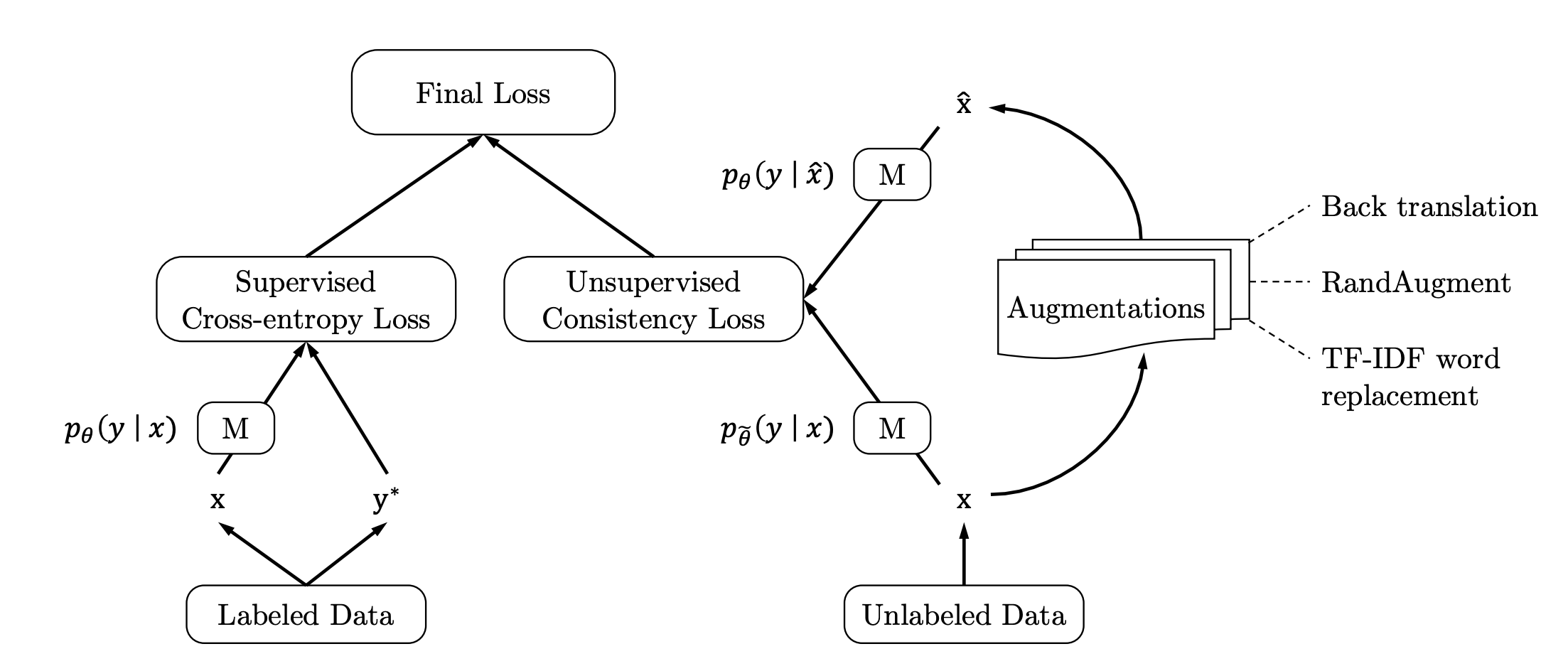The paper reports some spectacular results: using just 20 examples from IMDB, UDA gets an error-rate that surpasses BERT-large fine-tuned on the full 25k examples in the training set!

There was just one hitch: the Google implementation is in Python2 and Tensorflow v1 🤮Being allergic to both, we decided to see if we could reproduce the results from an open-source port to PyTorch. In hindsight, this turned out to be a foolish decision because now we were debugging against 3 frameworks! It was also a humbling lesson in not believing what is reported in some random repo you find on the internet 😉.

So in the end, I bit the bullet and decided to run Google's implementation which unsurprisingly worked out of the box.3 With just 10k steps and a few hours of training on a single GPU, UDA can indeed achieve > 90% accuracy on IMBD:

=== step 500 ===
INFO:tensorflow:  eval_classify_loss = 0.3957828
INFO:tensorflow:  eval_classify_accuracy = 0.57844
INFO:tensorflow:  loss = 0.80444646
=== step 1000 ===
INFO:tensorflow:  eval_classify_loss = 0.68793213
INFO:tensorflow:  eval_classify_accuracy = 0.56504
INFO:tensorflow:  loss = 1.4864826
=== step 2000 ===
INFO:tensorflow:  eval_classify_loss = 0.14758773
INFO:tensorflow:  eval_classify_accuracy = 0.89524
INFO:tensorflow:  loss = 0.71094906
=== step 10000 ===
INFO:tensorflow:  eval_classify_loss = 0.23858581
INFO:tensorflow:  eval_classify_accuracy = 0.91296
INFO:tensorflow:  loss = 0.23858581

So now that we're confident UDA really works, the next step will be to do a proper port to PyTorch - yay!

## TIL this week

1. Movement Pruning: Adaptive Sparsity by Fine-Tuning by Victor Sanh, Thomas Wolf, Alexander M. Rush (2020)

2. In a new term for me, this estimator is called straight-through because the top-$v$ function is ignored in the backward pass.

3. Well, almost. It took me a while to realise that when TensorFlow's TPUEstimator says it's running on a CPU, it's actually running on a GPU 🤷.